# Unit -III Chemical bonding.pptx

11. Jan 2023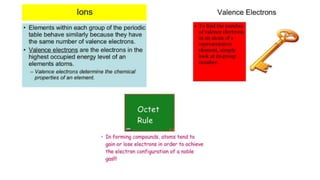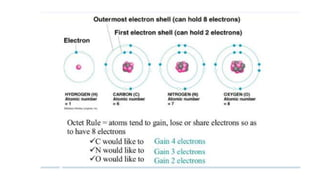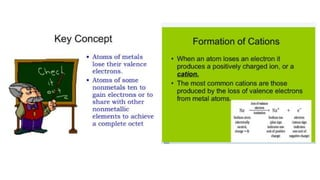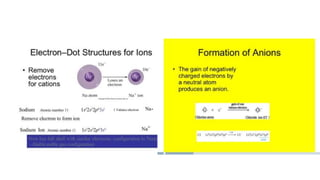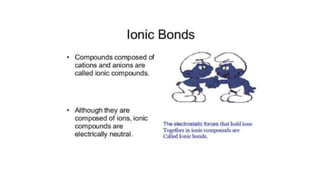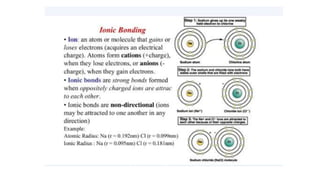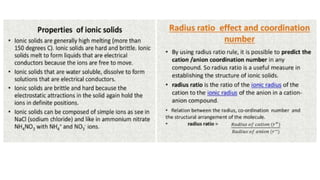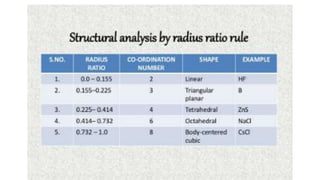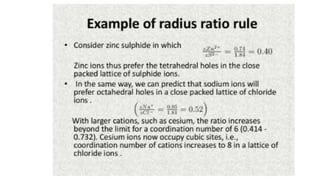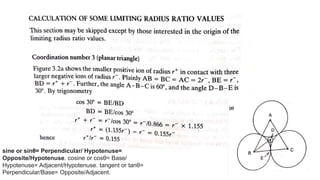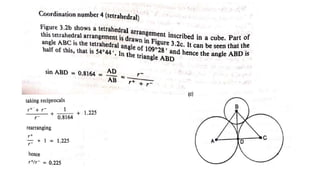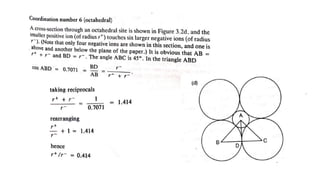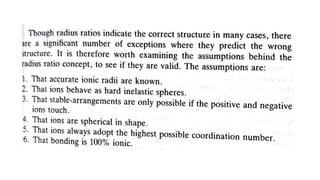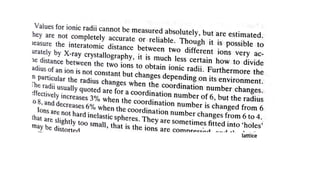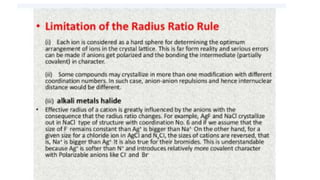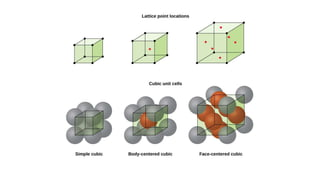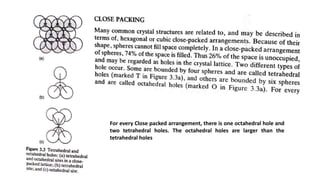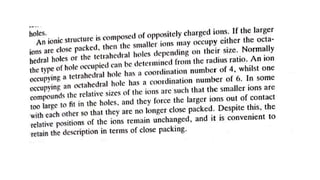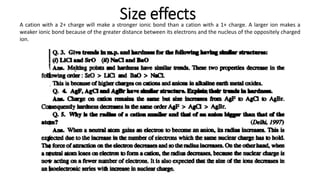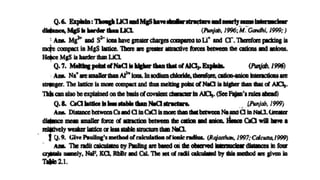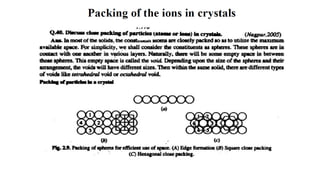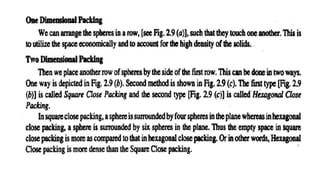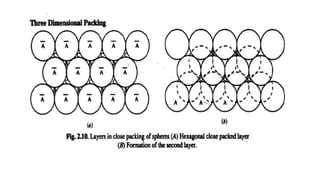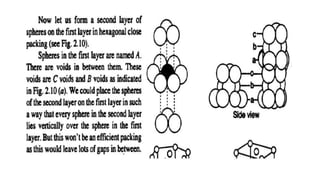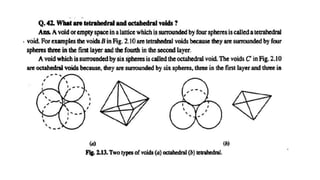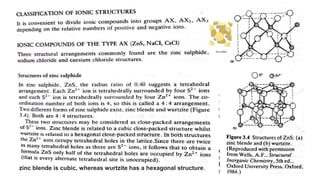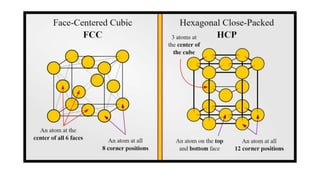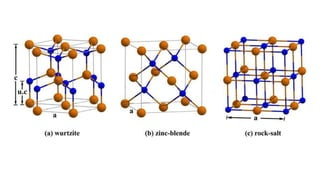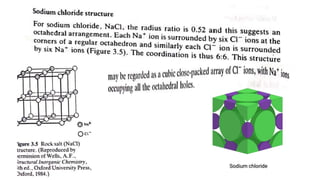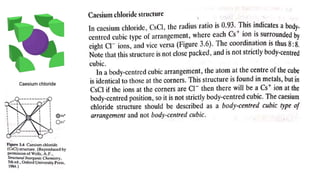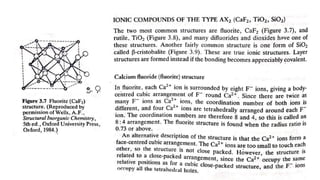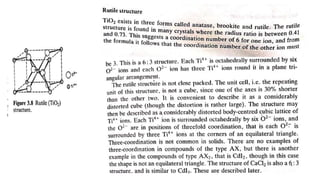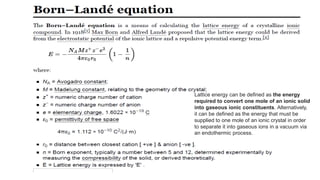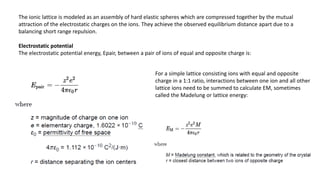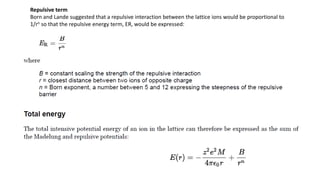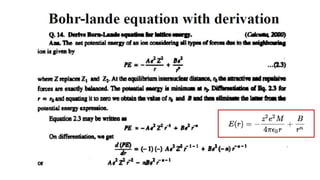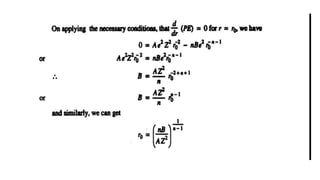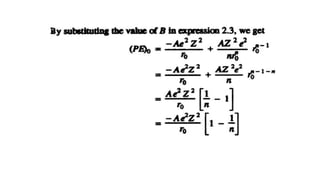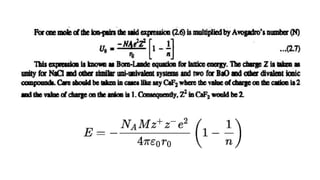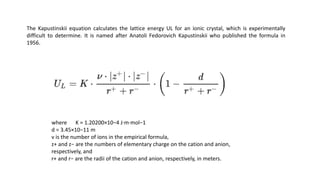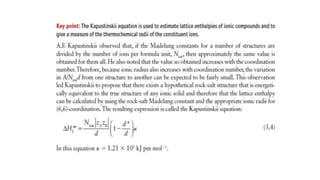1 von 42

### Unit -III Chemical bonding.pptx

• 1. Chemical Bonding Ionic bond and Covalent bond
• 11. sine or sinθ= Perpendicular/ Hypotenuse= Opposite/Hypotenuse. cosine or cosθ= Base/ Hypotenuse= Adjacent/Hypotenuse. tangent or tanθ= Perpendicular/Base= Opposite/Adjacent.
• 15. lattice
• 18. For every Close packed arrangement, there is one octahedral hole and two tetrahedral holes. The octahedral holes are larger than the tetrahedral holes
• 20. A cation with a 2+ charge will make a stronger ionic bond than a cation with a 1+ charge. A larger ion makes a weaker ionic bond because of the greater distance between its electrons and the nucleus of the oppositely charged ion.
• 27. zinc blende is cubic, whereas wurtzite has a hexagonal structure.
• 34. Lattice energy can be defined as the energy required to convert one mole of an ionic solid into gaseous ionic constituents. Alternatively, it can be defined as the energy that must be supplied to one mole of an ionic crystal in order to separate it into gaseous ions in a vacuum via an endothermic process.
• 35. The ionic lattice is modeled as an assembly of hard elastic spheres which are compressed together by the mutual attraction of the electrostatic charges on the ions. They achieve the observed equilibrium distance apart due to a balancing short range repulsion. Electrostatic potential The electrostatic potential energy, Epair, between a pair of ions of equal and opposite charge is: For a simple lattice consisting ions with equal and opposite charge in a 1:1 ratio, interactions between one ion and all other lattice ions need to be summed to calculate EM, sometimes called the Madelung or lattice energy:
• 36. Repulsive term Born and Lande suggested that a repulsive interaction between the lattice ions would be proportional to 1/rn so that the repulsive energy term, ER, would be expressed:
• 41. The Kapustinskii equation calculates the lattice energy UL for an ionic crystal, which is experimentally difficult to determine. It is named after Anatoli Fedorovich Kapustinskii who published the formula in 1956. where K = 1.20200×10−4 J·m·mol−1 d = 3.45×10−11 m ν is the number of ions in the empirical formula, z+ and z− are the numbers of elementary charge on the cation and anion, respectively, and r+ and r− are the radii of the cation and anion, respectively, in meters.